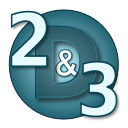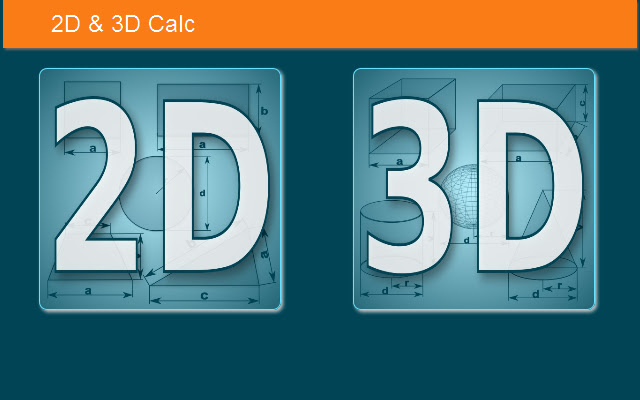# 2D & 3D Calc (delisted)

CRX id

## llgiihfjhohgmeikhnndplhhkhmmmgccDescription from extension meta

## Calculator for calculating circuit and area shapes, surface and volume solids.

Image from storeDescription from store Calculator for calculating perimeter (circumference) and area shapes, surface area and volume solids. Calculate: - perimeter of square and area of square - perimeter of rectangle and area of rectangle - circumference of circle and area of circle - perimeter of triangle and area of triangle - perimeter of rhombus and area of rhombus - perimeter of rhomboid and area of rhomboid - perimeter of trapezoid and area of trapezoid - circumference of ellipse and area of ellipse - surface area of cube and volume of cube - surface area of cuboid and volume of cuboid - surface area of sphere and volume of sphere - surface area of cylinder and volume of cylinder - surface area of cone and volume of cone Calculator is only for education.

### Latest reviews

• (2018-01-09) Максим: нет никакого калькулятора

Installs
1,000 history
Category
Rating